Normal view

# Infinite dimensional optimization and control theory

Material type:TextPublisher: Cambridge Cambridge University Press 1999Description: xv, 798 p. Includes bibliographical references and indexISBN: 9780521154543DDC classification: 003.5 Summary: This book is on existence and necessary conditions, such as Potryagin's maximum principle, for optimal control problems described by ordinary and partial differential equations. These necessary conditions are obtained from Kuhn–Tucker theorems for nonlinear programming problems in infinite dimensional spaces. The optimal control problems include control constraints, state constraints and target conditions. Evolution partial differential equations are studied using semigroup theory, abstract differential equations in linear spaces, integral equations and interpolation theory. Existence of optimal controls is established for arbitrary control sets by means of a general theory of relaxed controls. Applications include nonlinear systems described by partial differential equations of hyperbolic and parabolic type and results on convergence of suboptimal controls. https://www.cambridge.org/core/books/infinite-dimensional-optimization-and-control-theory/01A8F63A952B118229FB4BCE5BD01FD6#fndtn-information
Tags from this library: No tags from this library for this title.Average rating: 0.0 (0 votes)
Item type Current location Item location Collection Call number Status Date due Barcode
Books Vikram Sarabhai Library
General Stacks
Slot 16 (0 Floor, West Wing) Non-fiction 003.5 F2I6 (Browse shelf) Available 201670
##### Browsing Vikram Sarabhai Library shelves, Shelving location: General Stacks, Collection: Non-fiction Close shelf browser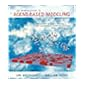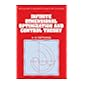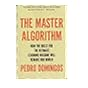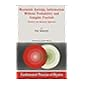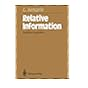003.3 A4 Agent-based modeling and simulation 003.3 M4I6 Introduction to mathematical modeling and computer simulations 003.3 W4I6 An introduction to agent based modeling: modeling natural, social, and engineered complex systems with netlogo 003.5 F2I6 Infinite dimensional optimization and control theory 003.54 D6M2 The master algorithm: how the quest for the ultimate learning machine will remake our world 003.54 J8M2 Maximum entropy information without probability and complex fractals: classical and quantum approach 003.54 J8R3 Relative information: theories and applications

Part I. Finite dimensional control problems
1. Calculus of variations and control theory
2. Optimal control problems without target conditions
3. Abstract minimization problems : the minimum principle for the time optimal problem
4. The minimum principle for general optimal control problems
Part II. Infinite dimensional control problems
5. Differential equations in Banach spaces and semigroup theory
6. Abstract minimization problems in Hilbert spaces
7. Abstract minimization problems in Banach spaces
8. Interpolation and domains of fractional powers
9. Linear control systems
10. Optimal control problems with state constraints
11. Optimal control problems with state constraints
Part III. Relaxed controls
12. Spaces of relaxed controls. Topology and measure theory
13. Relaxed controls in finite dimensional systems
14. Relaxed controls in infinite dimensional systems.

This book is on existence and necessary conditions, such as Potryagin's maximum principle, for optimal control problems described by ordinary and partial differential equations. These necessary conditions are obtained from Kuhn–Tucker theorems for nonlinear programming problems in infinite dimensional spaces. The optimal control problems include control constraints, state constraints and target conditions. Evolution partial differential equations are studied using semigroup theory, abstract differential equations in linear spaces, integral equations and interpolation theory. Existence of optimal controls is established for arbitrary control sets by means of a general theory of relaxed controls. Applications include nonlinear systems described by partial differential equations of hyperbolic and parabolic type and results on convergence of suboptimal controls.

https://www.cambridge.org/core/books/infinite-dimensional-optimization-and-control-theory/01A8F63A952B118229FB4BCE5BD01FD6#fndtn-information

There are no comments for this item.